# What is Rhombus?

• Last Updated : 27 Sep, 2021

In our daily lives, we come across a number of geometrical shapes and figures. These include construction of buildings, dams, interior design, computer graphics, etc. Geometry plays an important role in the study of as well as the creation of things around us. It is extensively used in various sciences, as well as architecture, sculpture, and art. All shapes such as rectangle, triangle, circle, square, cone, cylinder, etc. come under geometry. In other words, geometry is all about shapes and their properties. But the creation of new objects can be possible only through proper measurements so that the said objects are customized as per the needs of the people using them. For example, a water tank to be installed at someone’s rooftop must be of considerable size. It could be of the cylindrical or cubical shape, and its capacity(volume) must conform to the needs of the people using it, and also the amount of supply of water to that area. While these factors are considered, one also has to keep in mind the surface area that the tank would be taking up. This definitely calls for proper measurement and calculations before constructing and installing the tank. This is when the concept of mensuration comes into the picture.

### What is Mensuration?

Mensuration pertains to the computation of various dimensions of all the 2D and 3D shapes that we see around us. Not only that, but mensuration is also used to calculate the area, perimeter, lateral surface area, volume, etc. of various objects around us. All the calculations pertaining to geometrical shapes and sizes fall under this branch of mathematics.

### Types of Shapes: 2D and 3D

• A 2-Dimensional space is one that has only two dimensions, namely length, and breadth. Such shapes do not have any height or width. Their elemental position can be determined with the help of just two dimensions. We always determine the area or perimeter of such shapes. The figure below depicts some 2D figures: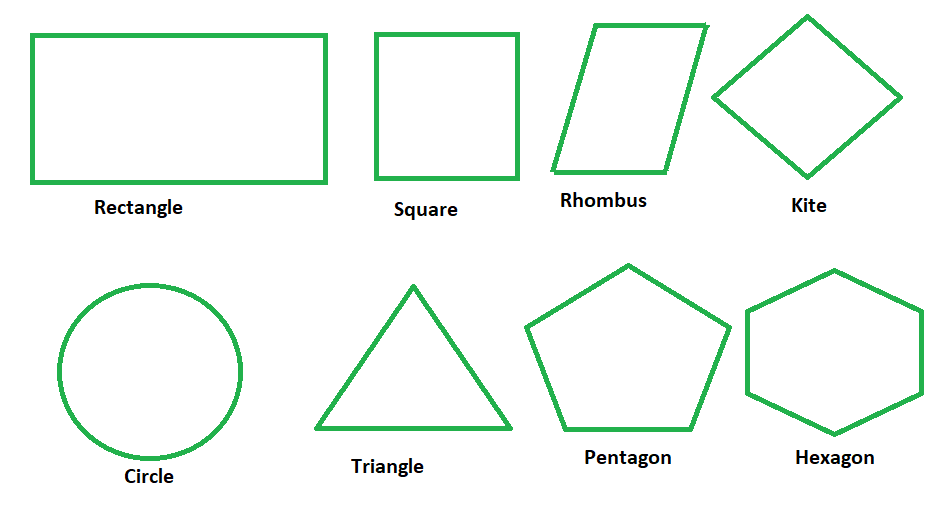• A 3-Dimensional space is one that has a height/width/depth, unlike 2D shapes. In the case of 3D shapes, volume, lateral surface area, the curved surface area is determined. Such shapes are made up of a number of 2D shapes themselves. The figure below depicts some 3D shapes: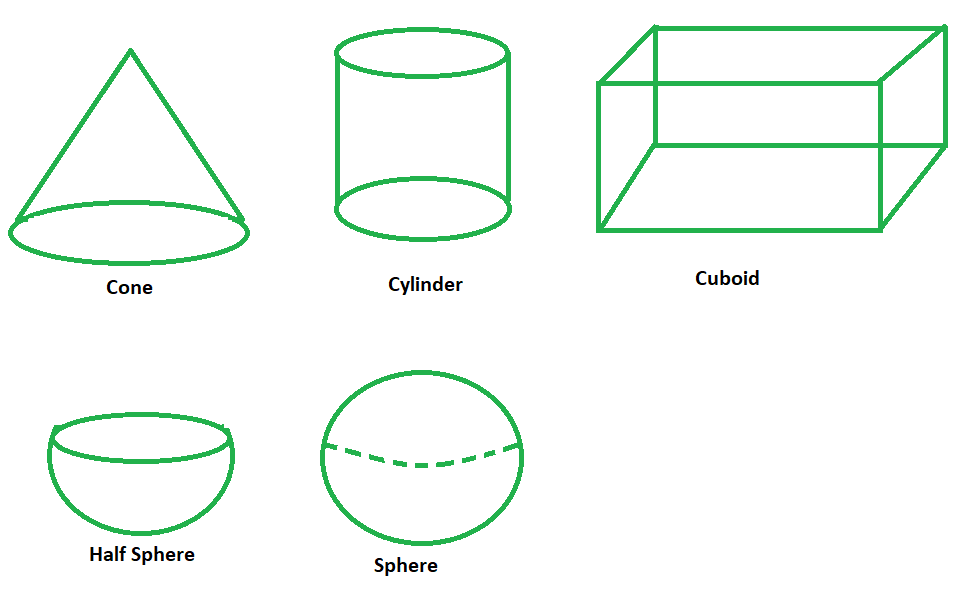### Basic Terminology

• Area: Such a quantity that displays the size/region/shape of a two-dimensional object. It can be found out by multiplying the length of such a shape by its breadth. The area can be calculated for two-dimensional shapes only. It is measured in square units.
• Perimeter: It is basically the distance around a shape. The sum of lengths of sides of a given shape is called its perimeter. In the case of ellipse/circle, the perimeter is called the circumference.
• Volume: It is a quantity pertaining to three-dimensional shapes. It depicts the shape that a 3-dimensional space/ object would occupy. Unlike area, it is measured in cubic units.
• Lateral Surface Area: The lateral surface of an object includes all sides of the object but excludes its base and top. Thus, the area of a shape that includes all the sides of such shape, but excludes its base and top is called its lateral surface area.
• Curved Surface Area: It is defined as the area of the curved surfaces of an object.
• Total Surface Area: It is defined as the total area of the surface occupied/excluded by a given object or shape. Unlike lateral and curved surface areas, it takes into consideration all the sides of the shape, be it the top/base/curves, etc.
• Diagonal: These are the line segments that are formed by joining two vertices of a quadrilateral (a geometrical shape formed by joining 4 points).

A quadrilateral is a geometrical shape that is formed by joining four points, three of which must be non-collinear, i.e., must not lie in the same straight line. If that is the case, then the points conjoined would form a straight line instead of a shape. Thus it is essential that a quadrilateral has four edges and four vertices. Hence such a polygon that has four sides and four angles is called a quadrilateral. The sum of interior angles of a quadrilateral must always be equal to 360°. It is imperative to note that the quadrilaterals being discussed here are all 2D shapes only. Not that a quadrilateral cannot be a 3D shape. In 3 dimensional geometry, quadrilaterals need not be as plain as they are in 2-dimensional geometry. They can be folded along a diagonal, so it does not necessitate them to have vertices that share the same plane.

The following figure depicts various quadrilateral shapes in 2-dimensional geometry: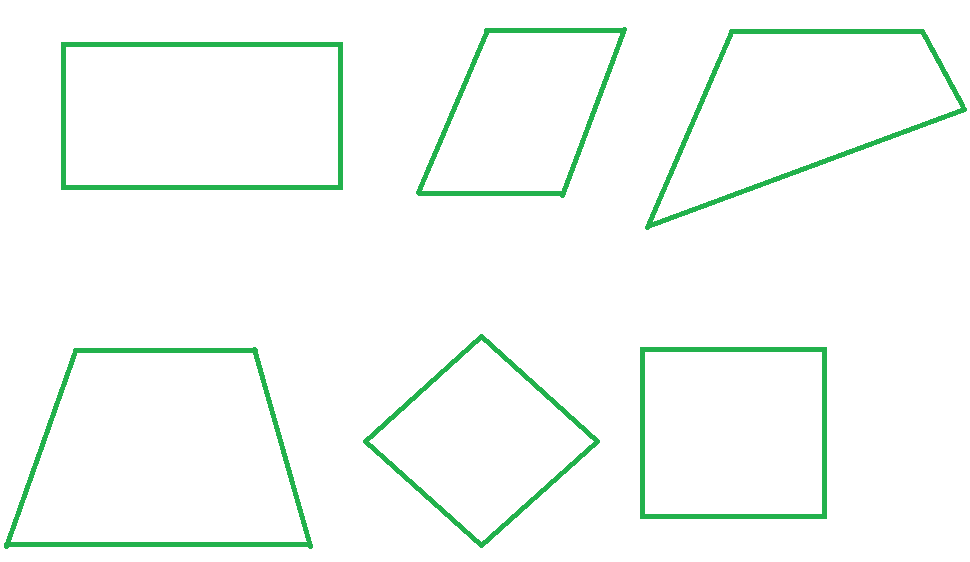We notice how all those shapes have 4 sides and vertices. All the above polygons can be categorized as quadrilaterals. Now, based on their different properties, be it on the lines of length of sides, or intersection of diagonals, etc. quadrilaterals are divided into various kinds. Based on such division, we have certain types/kinds of quadrilaterals that can be distinguished from each other to make their properties more clear. This is discussed in the next section.

• Square: Such a quadrilateral whose all sides are equal and all four angles measure 90°. It is a rectangle whose adjacent sides are equal, implying all four sides are equal in length. The figure below shows a square ABCD, where AB = BD = DC = CA. Also, ∠CAB = ∠ABD = ∠BDC = ∠ DCA = 90°.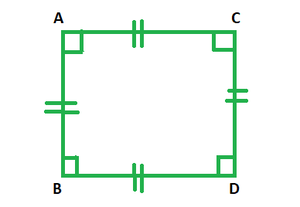• Rectangle: Such a quadrilateral has all angles which measure 90 degrees and whose parallel sides are equal. Such a rectangle forms a square when its adjacent sides are equal. The figure below depicts a rectangle ABCD, whose angles are equal to 90 degrees each and the parallel sides are equal in length.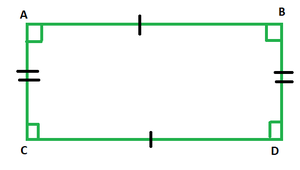• Parallelogram: Such a quadrilateral that has two pairs of parallel sides. Such opposite sides of a parallelogram are equal in length. The diagonals of a parallelogram bisect each other. As shown in the figure below, AC and BD are the two diagonals bisecting each other, and parallel sides are equal to each other.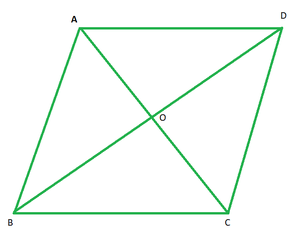• Rhombus: Such a special case of parallelogram where the adjacent sides are equal in length and also the diagonals bisect each other at right angles. In the figure below, we can see that AB = BC = CD = DA. Also, the diagonals AC and BD bisect each other at a right angle.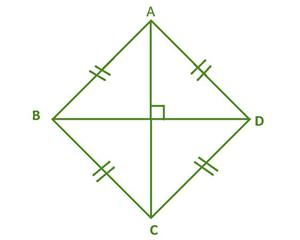• Trapezium: Such a quadrilateral that has at least one pair of parallel sides is called a trapezium. As depicted in the figure below, ABCD is a trapezium, with AB and CD being parallel to each other.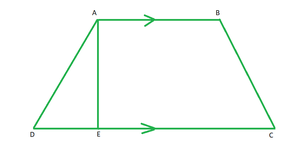Having discussed all these kinds, it is time to study rhombus at length now.

### What is a Rhombus?

A rhombus is a special case of parallelogram where the adjacent sides are equal in length and also the diagonals bisect each other at right angles. We can also state that a rhombus is actually a square only when all its angles are equal to 90 degrees. Since in a square, all four sides are equal, angles measure 90 degrees each and diagonals bisect at right angles as well. In the figure below, we can see that AB = BC = CD = DA. Also, the diagonals AC and BD bisect each other at a right angle.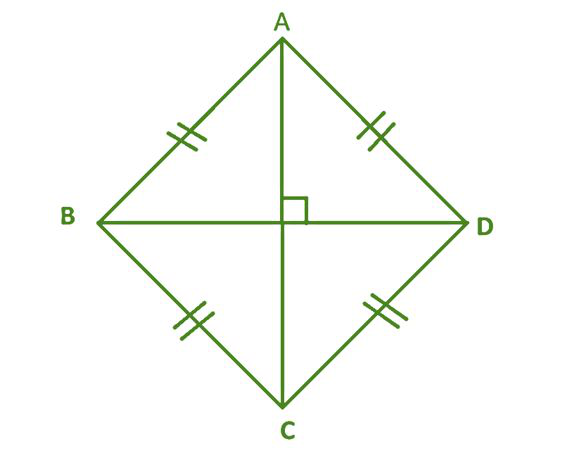Diagonals of a Rhombus

All rhombuses have two diagonals, which connect the pairs of opposite vertices. A rhombus is symmetrical along both its diagonals. The diagonals of a rhombus are perpendicular and bisect at right angles.

Properties of a Rhombus

• All the sides of a rhombus are equal. In fact, it is just a parallelogram with equal adjacent sides.
• A rhombus has opposite sides that are parallel to each other.
• The opposite angles of a rhombus are equal to each other.
• In the event of all the angles of a rhombus being equal, it is called a square.
• The diagonals of a rhombus would always bisect each other at a 90 degrees angle.
• Not only do the diagonals bisect each other, but they also bisect the angles of a rhombus.
• The two diagonals of a rhombus divide it into four right-angled congruent triangles.
• Two adjacent angles of a rhombus add up to 180 degrees.
• There cannot be a circumscribing circle around a rhombus.
• It is impossible to have an inscribing circle inside a rhombus.
• By joining the midpoints of the four sides together, a rectangle can be formed. Such a rectangle would have half the length and width to that of the length of the diagonals. As such, the area of this rectangle would be half as much as the area of the rhombus. This is illustrated in the figure below: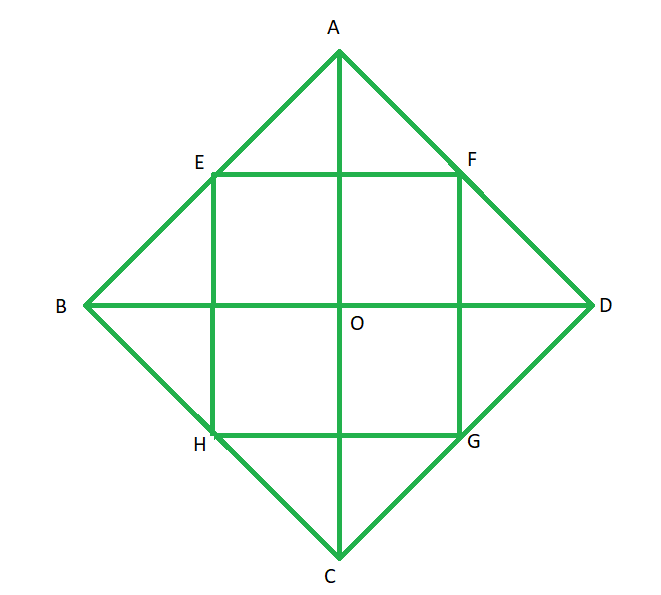• Area of a rhombus is equal to half the product of the lengths of both diagonals. Hence, if p and q denote the lengths of diagonals of a rhombus, its area = 1/2 × p × q.
• Perimeter of a rhombus is defined as the sum of all its sides. Since all the sides of a rhombus are equal in length, it can be said that the perimeter of a rhombus is four times the length of one side. Thus, if h denotes the length of a side of rhombus, its perimeter = 4h.

### Conceptual Questions

Question 1. Assume quadrilateral MNOP is a rhombus. If diagonal MO = 29 and diagonal NP = 14, what is the area of rhombus MNOP.

Solution:

We know, Area of a rhombus = (d1)(d2)/2

Substituting the lengths of diagonals in the above formula, we have:

A = (29)(14)/2 = 406/2 = 203

The area of rhombus MNOP is therefore 203 square units.

Question 2. Assume quadrilateral ABCD is a rhombus. The perimeter of ABCD is 40, and the length of one of its diagonals is 12. What is the area of ABCD?

Solution:

Since the perimeter of the rhombus is 40, and by definition all 4 sides of a rhombus have the same length, we know that the length of each side is 10. We can find the length of the other diagonal if we recognize that the two diagonals combined with a side edge form a right triangle. The length of the hypotenuse is 10, and each leg of the triangle is equal to one-half of each diagonal. We can therefore set up an equation involving Pythagorean’s Theorem as follows:

102 = x2 + 62, where x is equal to one-half the length of the unknown diagonal.

We can therefore solve for x as follows:

x2 = 102 − 62 = 100 − 36 = 64

x is therefore equal to 8, and our other diagonal is 16. We can now use both diagonals to solve for the area of the rhombus:

A = (16)(12)/ 2 = 192/ 2 = 96.

The area of rhombus ABCD is therefore equal to 96 square units.

Question 3. Find the area of a rhombus with diagonal lengths of (2x+2) and (4x+4) units.

Solution:

We know, Area of a rhombus = (d1)(d2)/2

Substituting the lengths of diagonals in the above formula, we have:

A == (4x2 + 8x + 4) in2

Question 4. Find the area of a rhombus if its diagonal lengths arecm andcm.

Solution:

We know, Area of a rhombus = (d1)(d2)/2

Substituting the lengths of diagonals in the above formula, we have:

A =cm2

My Personal Notes arrow_drop_up